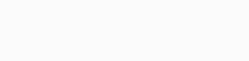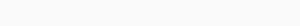#Quants Questions : Simplification Set 4

Hello Aspirants. Welcome to Online Quantitative Aptitude Section with explanation in AffairsCloud.com. Here we are creating question sample in Simplification ,which is common for all the  competitive exams like SSC CHSL,SSC CGL,FCI EXAM .We have included Some questions that are repeatedly asked in bank exams !!!This is simplification quiz for RBI assistant exam 2015.

1. 78×(1024)1/2÷ ? = 208
A)11
B)12
C)13
D)14
Explanation :
X = (78×32) / 208 = 2496/208 = 12

2. (4×12÷16× 27) ÷ 3?   =  1
A)2
B)3
C)4
D)5
Explanation :3. (1/3) of (5/7) of (5/11) of ? = 325
A)3003
B)3030
C)3300
D)3001
Explanation :4. 345.7 + 852.5 – 108.4 = 436.7 + ?
A)653.0
B)653.1
C)653.2
D)653.3
Explanation :
1198.2 – 108.4 = 436.7 + ?
1089.8 = 436.7+653.1

5. 20 % 650 + 150 %  ? =  2005
A)1254
B)1253
C)1251
D)1250
Explanation :
130 + (150x/100) = 2005
2005 – 130 = 150x/100
3x/2 = 1875
X = (1875×2)/3 = 1250

6. (3/2) + (8/7) ×(42/5) ÷ (4/3) = ?
A)9.5
B)8.7
C)9.2
D)8.6
Explanation :7. X2 + (3025)1/2  = 3899
A)60
B)61
C)62
D)63
Explanation :X2 + 55 = 3899
X2 = 3899 – 55
X2 =3844
X = 62

8.A)358
B)357
C)356
D)355
Explanation :
5×49×1.46 = 357.7 = 358

9. 11.001 × ? × 25.875 = 678543
A)2380
B)2381
C)2382
D)2383
Explanation :
? =678543 / (11.001 ×25.875)
? = 678543 / 284.7
? = 2383

10. (15.02)2 – 1232.08 = ?
A)1006.4
B)-1006.4
C)1004.6
D)-1004.6